Скачать презентацию Mean-Reverting Models in Financial and Energy Markets Anatoliy

ba4f7abf1e222521fa9bb59f94db32f7.ppt

• Количество слайдов: 66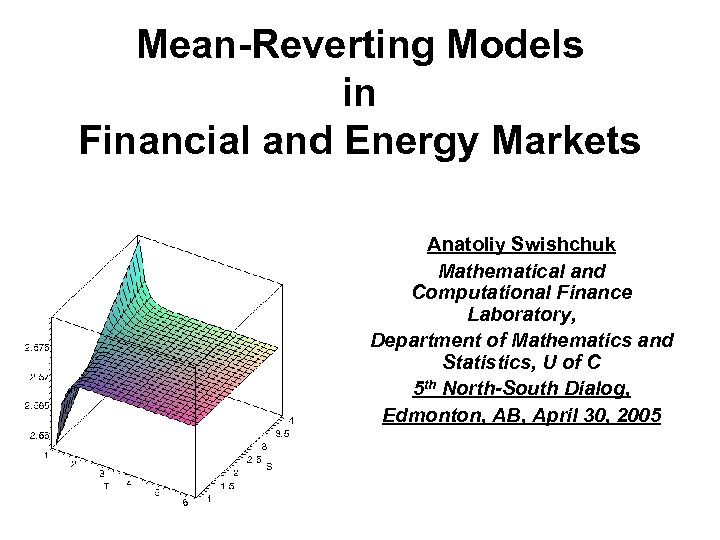Mean-Reverting Models in Financial and Energy Markets Anatoliy Swishchuk Mathematical and Computational Finance Laboratory, Department of Mathematics and Statistics, U of C 5 th North-South Dialog, Edmonton, AB, April 30, 2005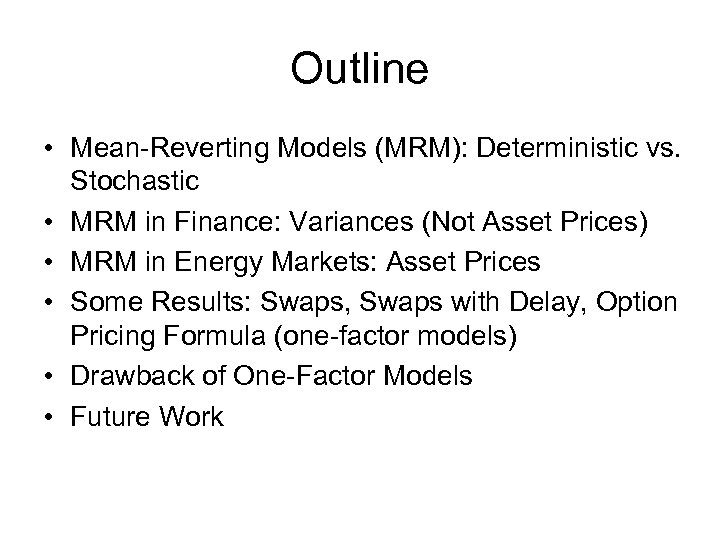Outline • Mean-Reverting Models (MRM): Deterministic vs. Stochastic • MRM in Finance: Variances (Not Asset Prices) • MRM in Energy Markets: Asset Prices • Some Results: Swaps, Swaps with Delay, Option Pricing Formula (one-factor models) • Drawback of One-Factor Models • Future Work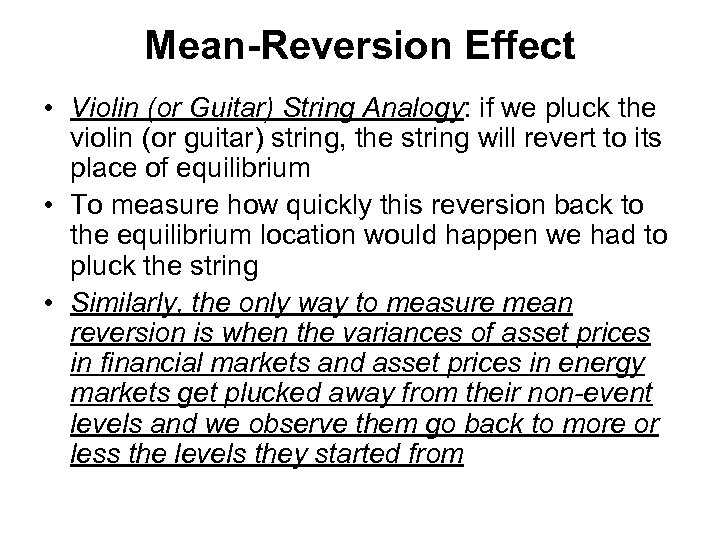Mean-Reversion Effect • Violin (or Guitar) String Analogy: if we pluck the violin (or guitar) string, the string will revert to its place of equilibrium • To measure how quickly this reversion back to the equilibrium location would happen we had to pluck the string • Similarly, the only way to measure mean reversion is when the variances of asset prices in financial markets and asset prices in energy markets get plucked away from their non-event levels and we observe them go back to more or less the levels they started from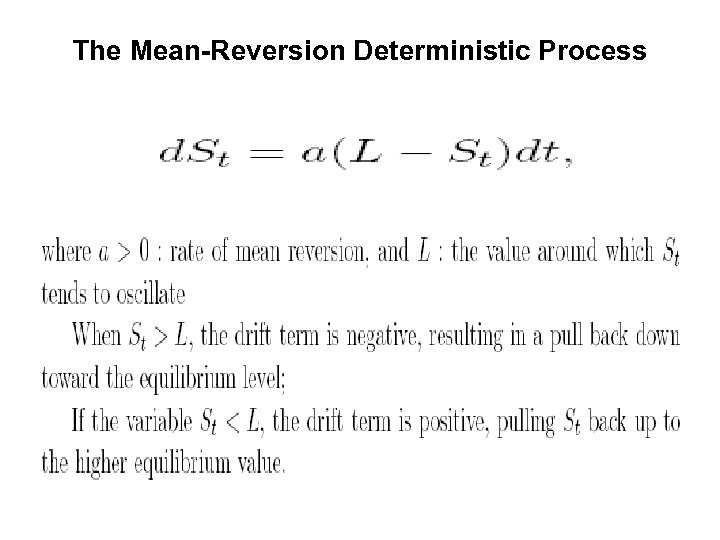The Mean-Reversion Deterministic Process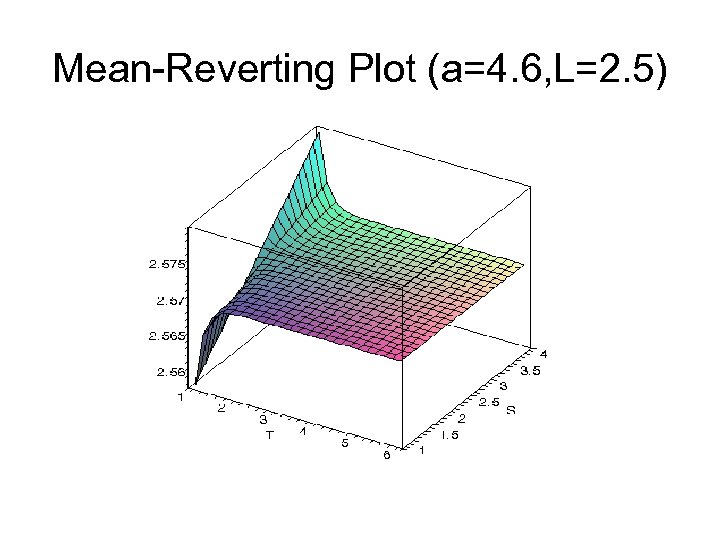Mean-Reverting Plot (a=4. 6, L=2. 5)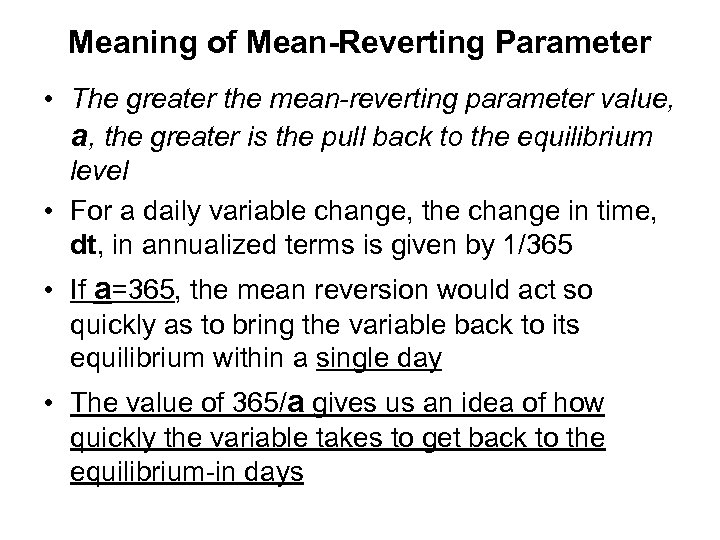Meaning of Mean-Reverting Parameter • The greater the mean-reverting parameter value, a, the greater is the pull back to the equilibrium level • For a daily variable change, the change in time, dt, in annualized terms is given by 1/365 • If a=365, the mean reversion would act so quickly as to bring the variable back to its equilibrium within a single day • The value of 365/a gives us an idea of how quickly the variable takes to get back to the equilibrium-in days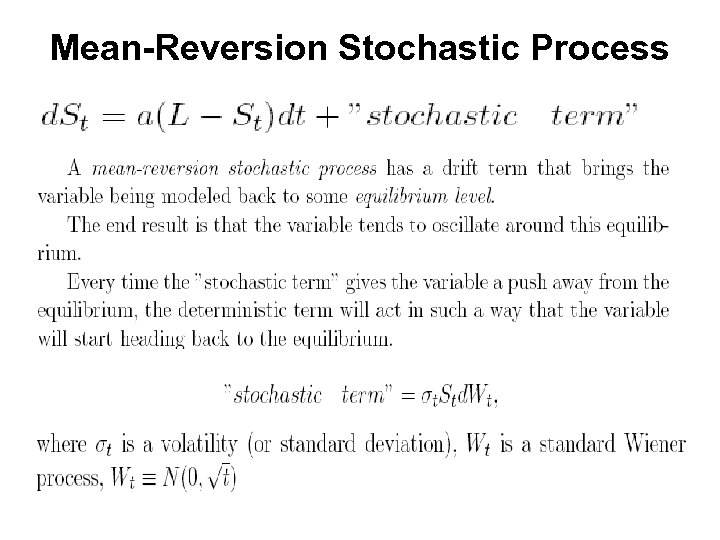Mean-Reversion Stochastic Process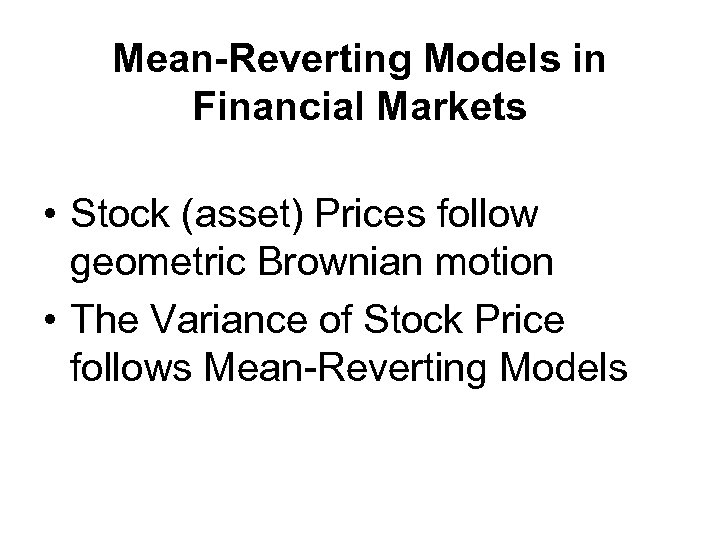Mean-Reverting Models in Financial Markets • Stock (asset) Prices follow geometric Brownian motion • The Variance of Stock Price follows Mean-Reverting Models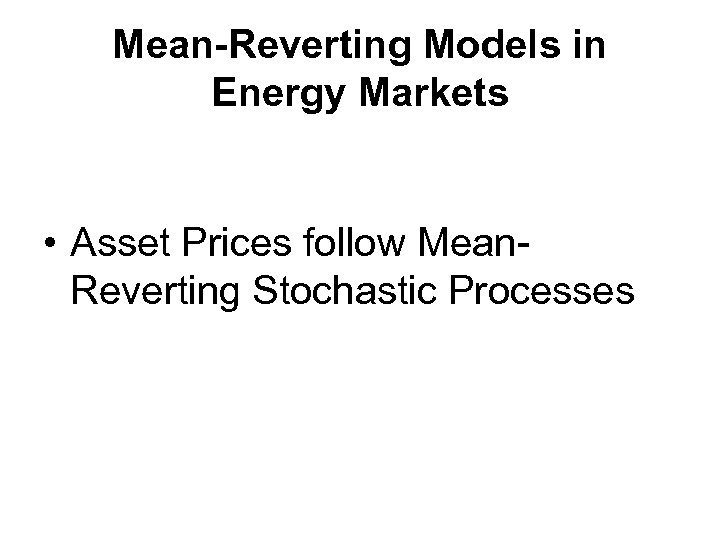Mean-Reverting Models in Energy Markets • Asset Prices follow Mean. Reverting Stochastic Processes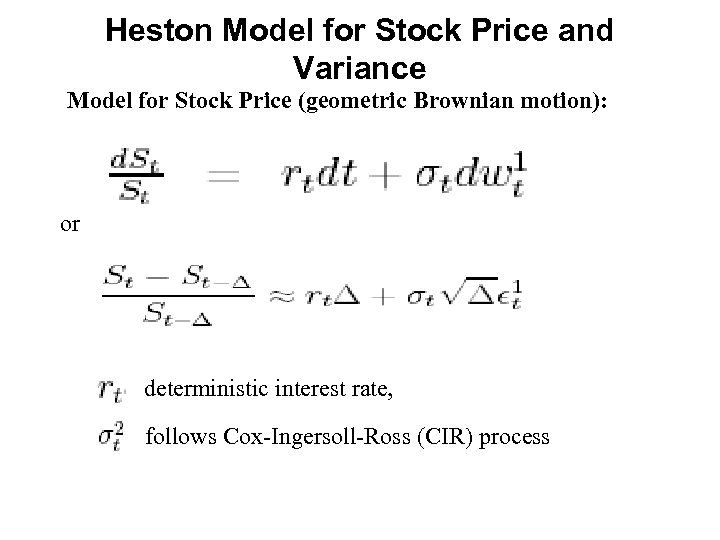Heston Model for Stock Price and Variance Model for Stock Price (geometric Brownian motion): or deterministic interest rate, follows Cox-Ingersoll-Ross (CIR) process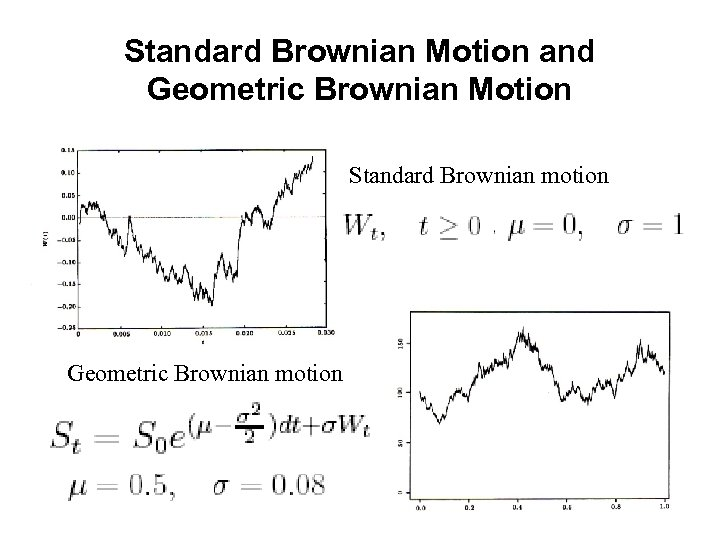Standard Brownian Motion and Geometric Brownian Motion Standard Brownian motion Geometric Brownian motion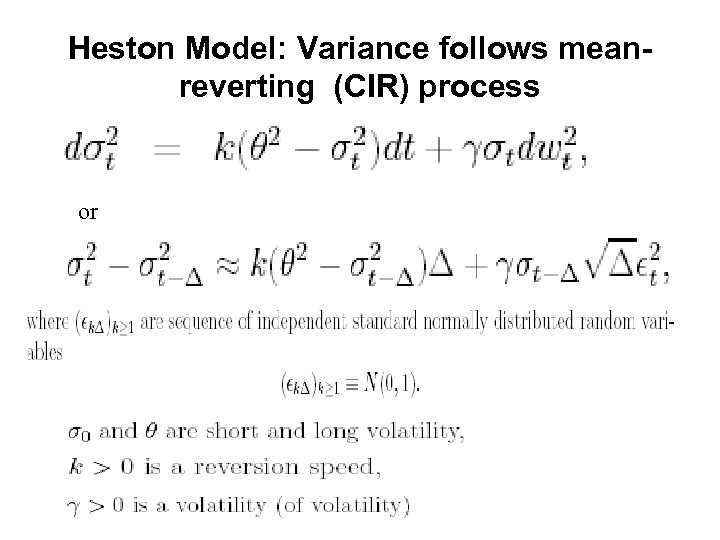Heston Model: Variance follows meanreverting (CIR) process or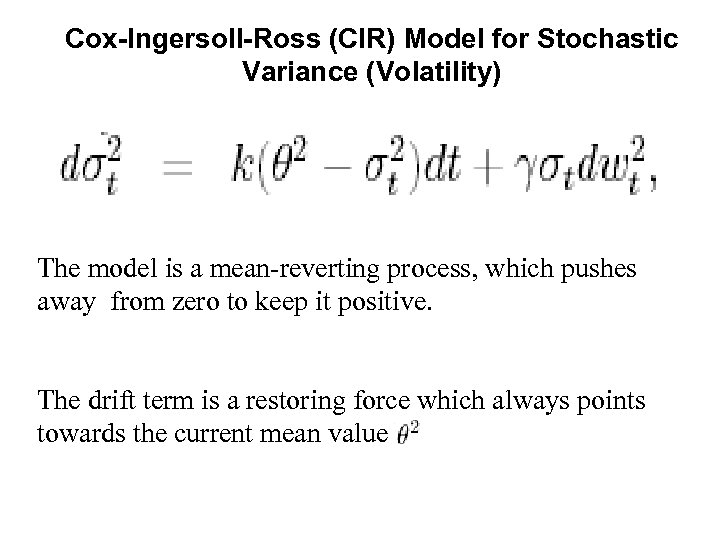Cox-Ingersoll-Ross (CIR) Model for Stochastic Variance (Volatility) The model is a mean-reverting process, which pushes away from zero to keep it positive. The drift term is a restoring force which always points towards the current mean value.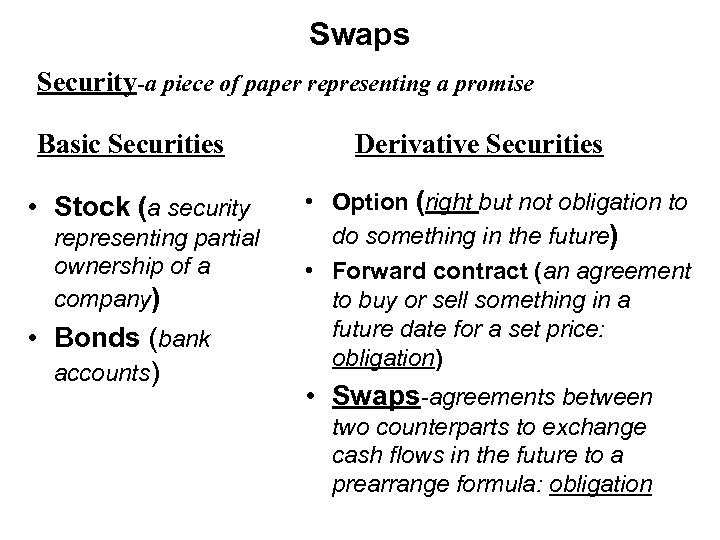Swaps Security-a piece of paper representing a promise Basic Securities • Stock (a security representing partial ownership of a company) • Bonds (bank accounts) Derivative Securities • Option (right but not obligation to do something in the future) • Forward contract (an agreement to buy or sell something in a future date for a set price: obligation) • Swaps-agreements between two counterparts to exchange cash flows in the future to a prearrange formula: obligation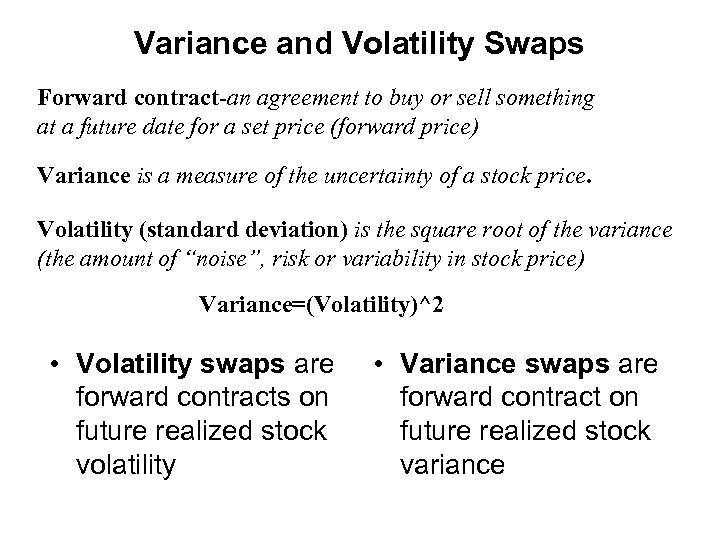Variance and Volatility Swaps Forward contract-an agreement to buy or sell something at a future date for a set price (forward price) Variance is a measure of the uncertainty of a stock price. Volatility (standard deviation) is the square root of the variance (the amount of “noise”, risk or variability in stock price) Variance=(Volatility)^2 • Volatility swaps are forward contracts on future realized stock volatility • Variance swaps are forward contract on future realized stock variance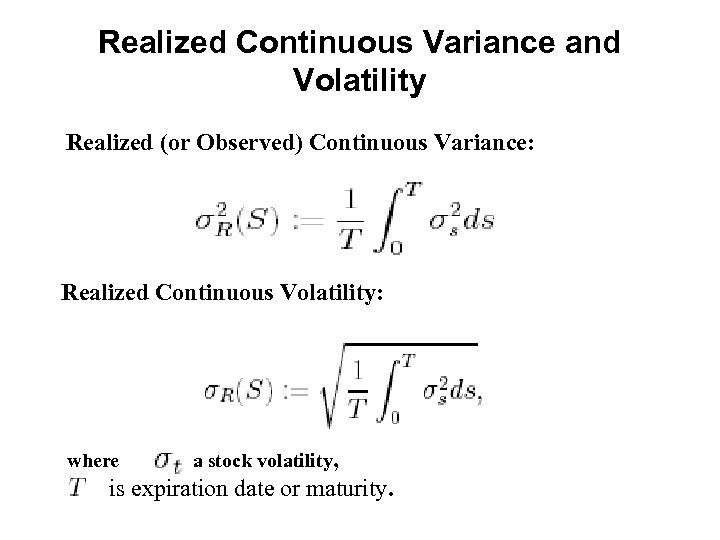Realized Continuous Variance and Volatility Realized (or Observed) Continuous Variance: Realized Continuous Volatility: where is a stock volatility, is expiration date or maturity.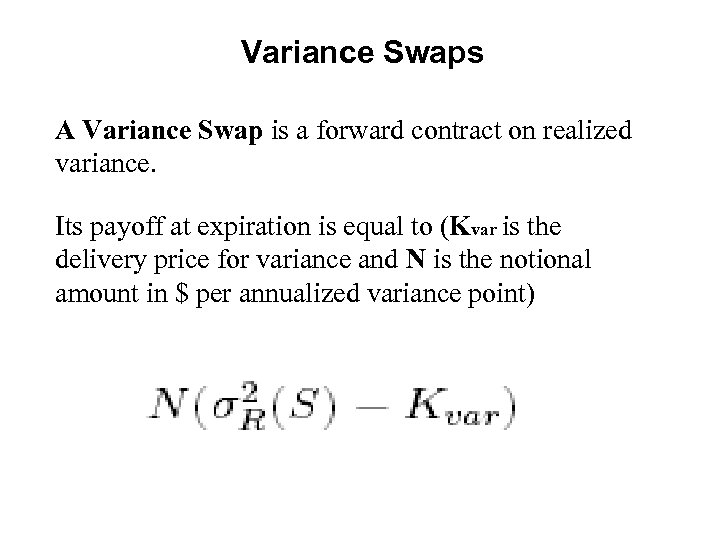Variance Swaps A Variance Swap is a forward contract on realized variance. Its payoff at expiration is equal to (Kvar is the delivery price for variance and N is the notional amount in \$ per annualized variance point)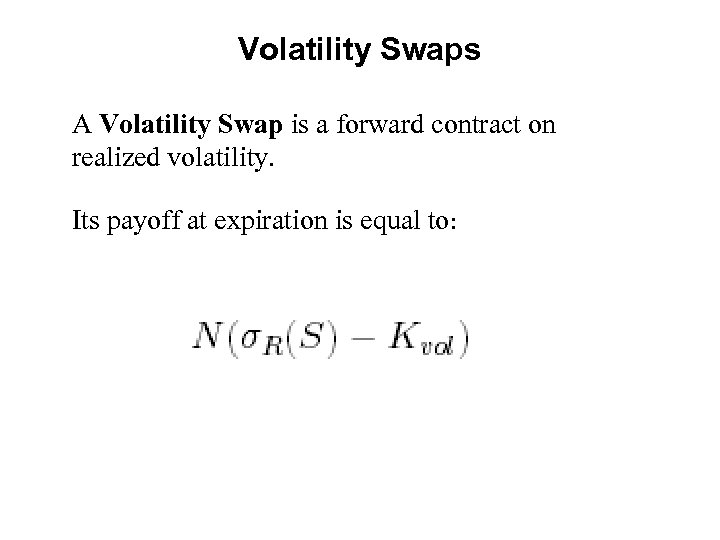Volatility Swaps A Volatility Swap is a forward contract on realized volatility. Its payoff at expiration is equal to: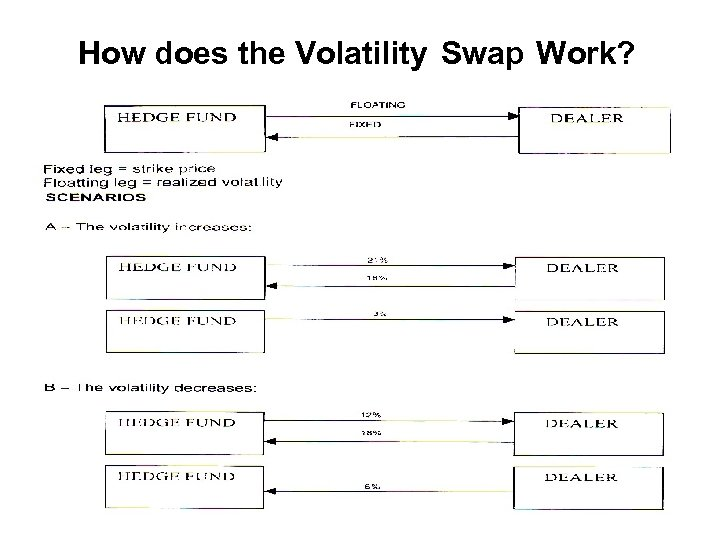How does the Volatility Swap Work?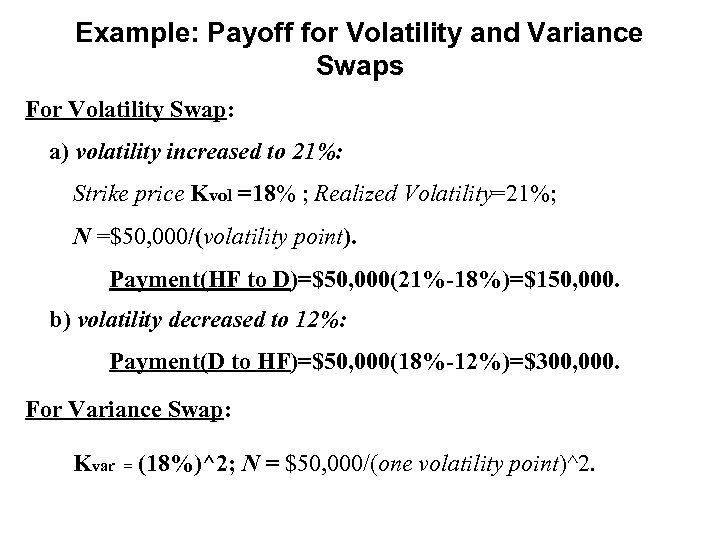Example: Payoff for Volatility and Variance Swaps For Volatility Swap: a) volatility increased to 21%: Strike price Kvol =18% ; Realized Volatility=21%; N =\$50, 000/(volatility point). Payment(HF to D)=\$50, 000(21%-18%)=\$150, 000. b) volatility decreased to 12%: Payment(D to HF)=\$50, 000(18%-12%)=\$300, 000. For Variance Swap: Kvar = (18%)^2; N = \$50, 000/(one volatility point)^2.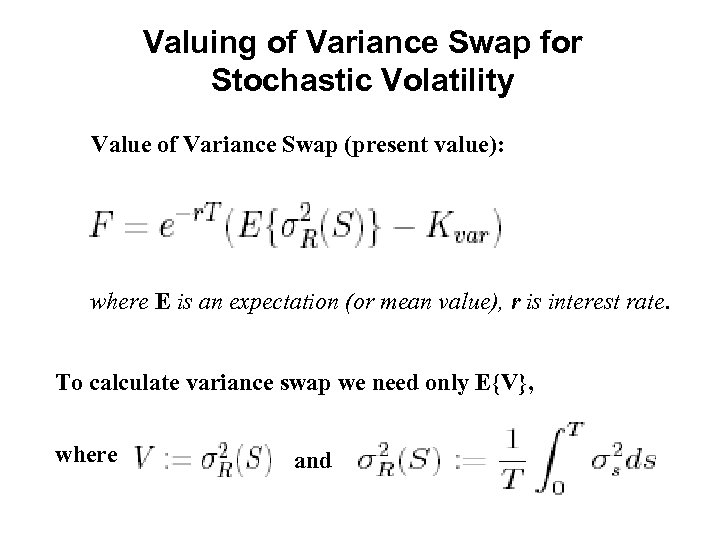Valuing of Variance Swap for Stochastic Volatility Value of Variance Swap (present value): where E is an expectation (or mean value), r is interest rate. To calculate variance swap we need only E{V}, where and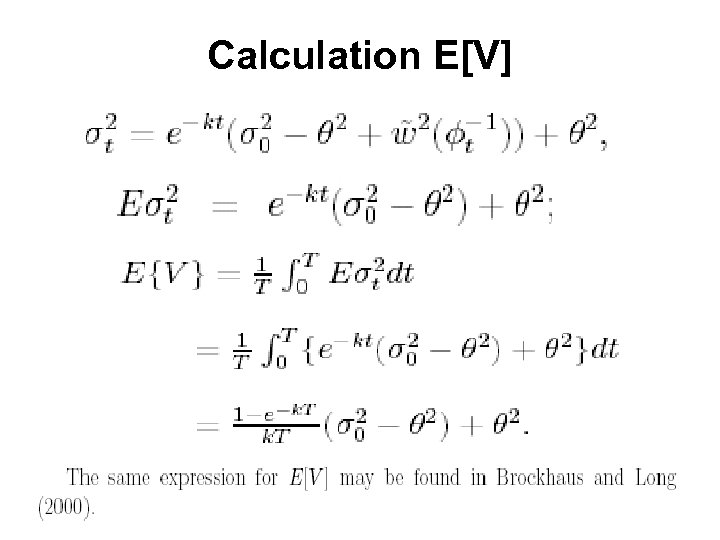Calculation E[V]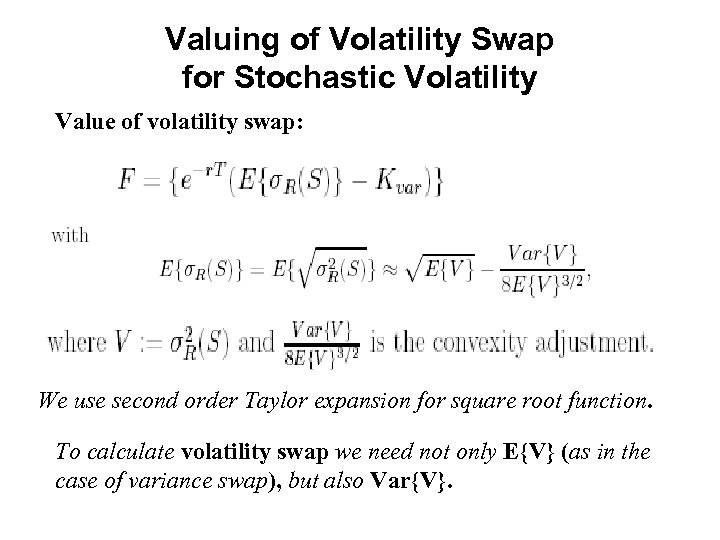Valuing of Volatility Swap for Stochastic Volatility Value of volatility swap: We use second order Taylor expansion for square root function. To calculate volatility swap we need not only E{V} (as in the case of variance swap), but also Var{V}.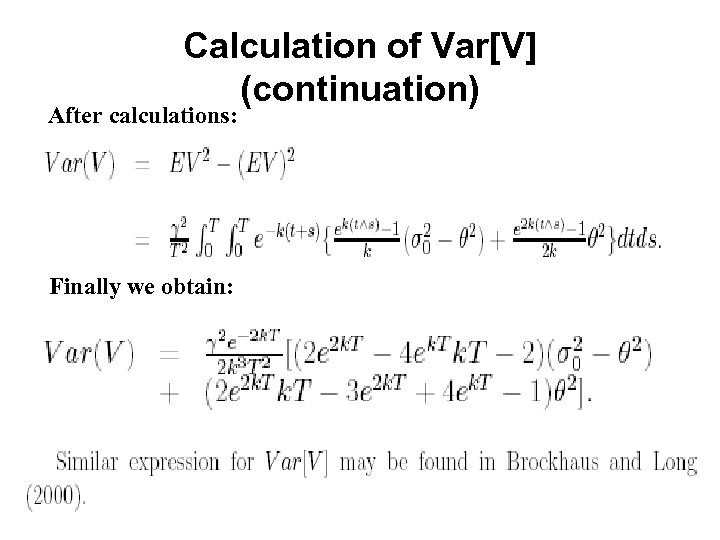Calculation of Var[V] (continuation) After calculations: Finally we obtain: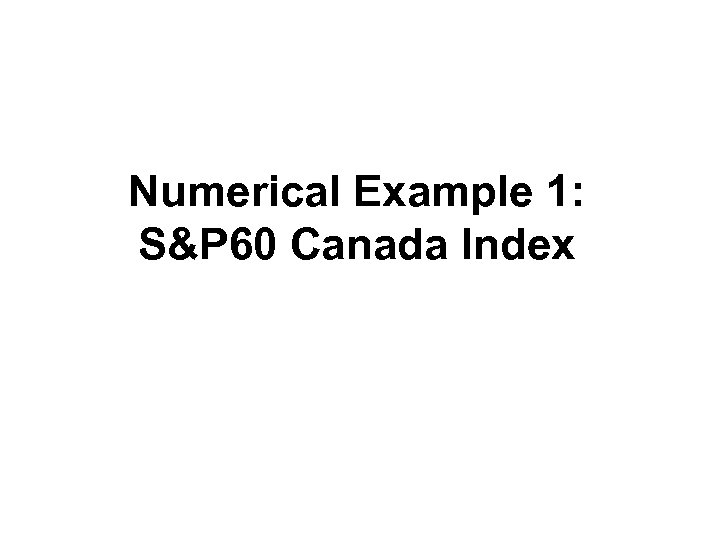Numerical Example 1: S&P 60 Canada Index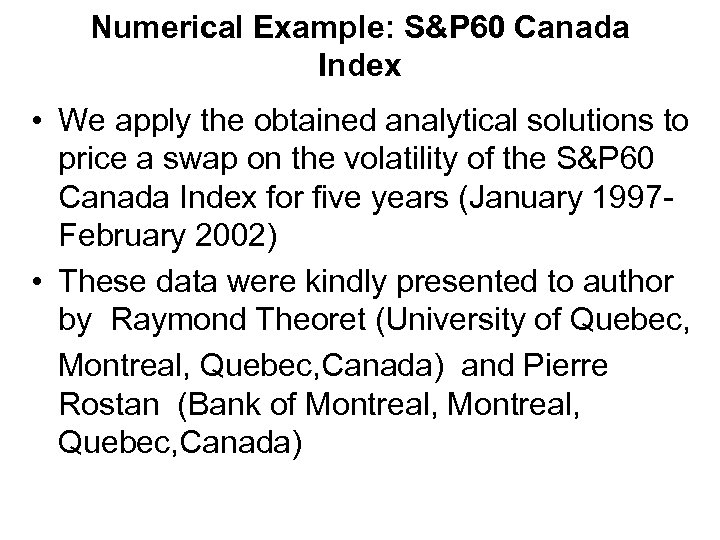Numerical Example: S&P 60 Canada Index • We apply the obtained analytical solutions to price a swap on the volatility of the S&P 60 Canada Index for five years (January 1997 February 2002) • These data were kindly presented to author by Raymond Theoret (University of Quebec, Montreal, Quebec, Canada) and Pierre Rostan (Bank of Montreal, Quebec, Canada)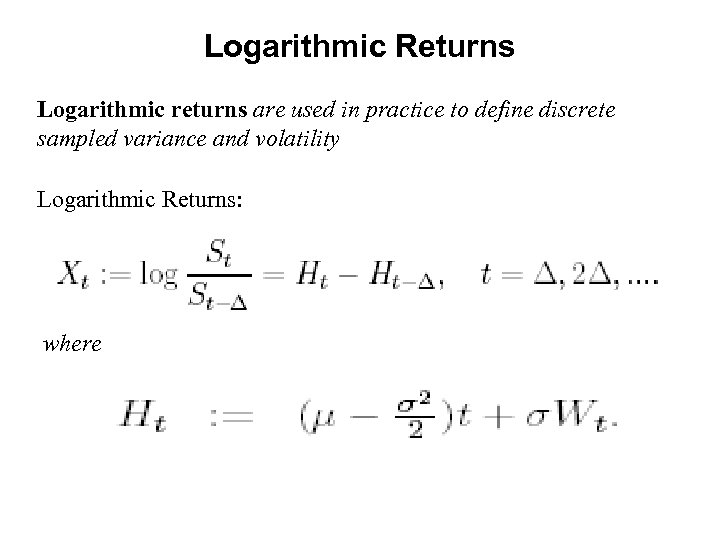Logarithmic Returns Logarithmic returns are used in practice to define discrete sampled variance and volatility Logarithmic Returns: where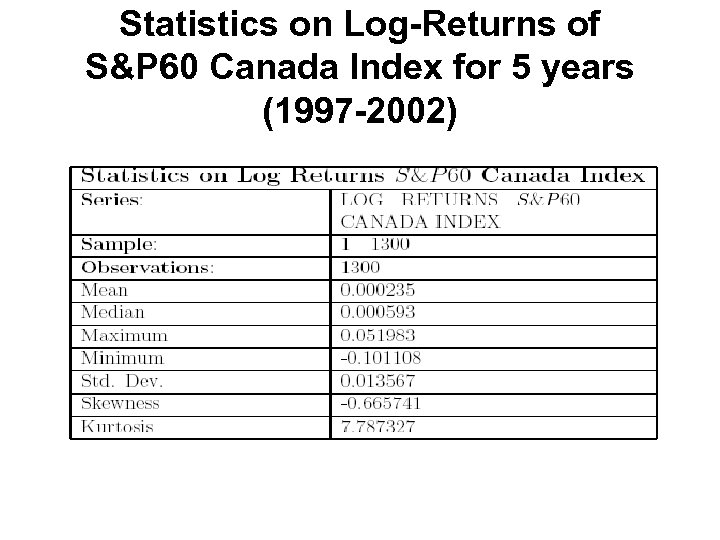Statistics on Log-Returns of S&P 60 Canada Index for 5 years (1997 -2002)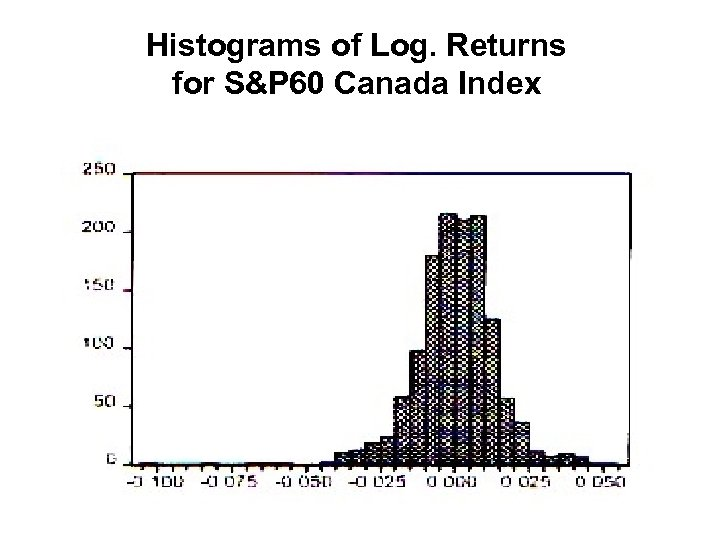Histograms of Log. Returns for S&P 60 Canada Index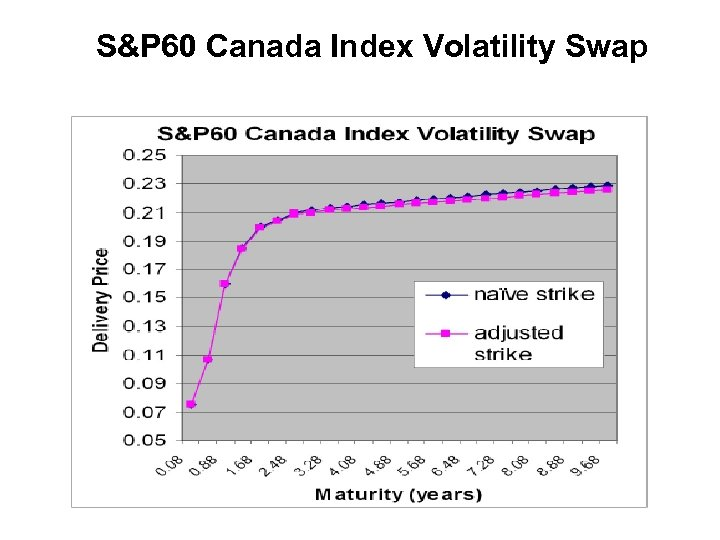S&P 60 Canada Index Volatility Swap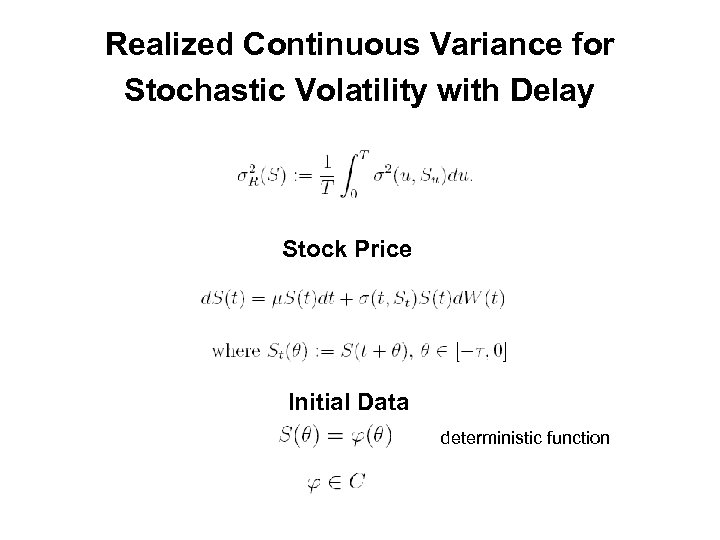Realized Continuous Variance for Stochastic Volatility with Delay Stock Price Initial Data deterministic function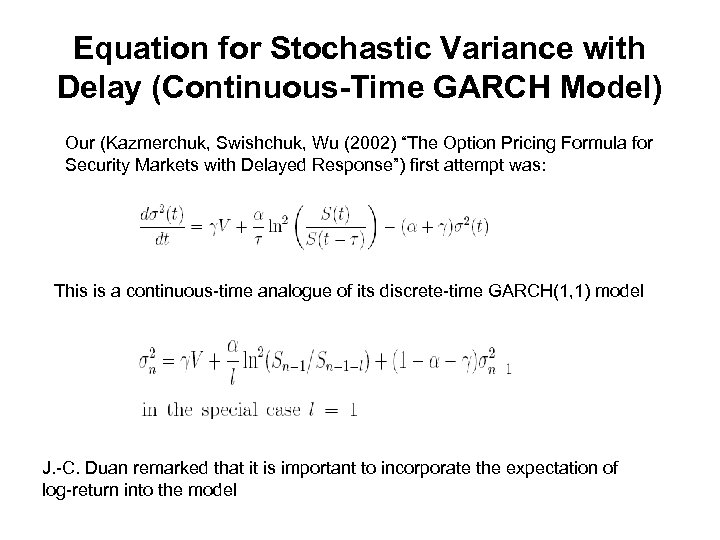Equation for Stochastic Variance with Delay (Continuous-Time GARCH Model) Our (Kazmerchuk, Swishchuk, Wu (2002) “The Option Pricing Formula for Security Markets with Delayed Response”) first attempt was: This is a continuous-time analogue of its discrete-time GARCH(1, 1) model J. -C. Duan remarked that it is important to incorporate the expectation of log-return into the model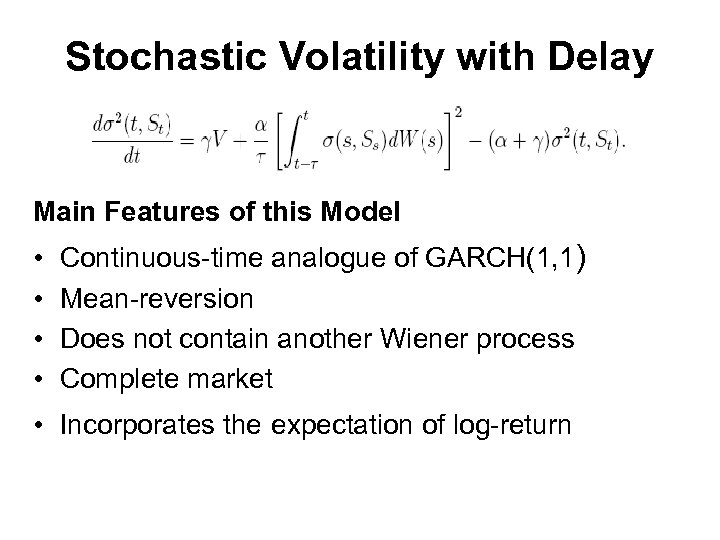Stochastic Volatility with Delay Main Features of this Model • • Continuous-time analogue of GARCH(1, 1) Mean-reversion Does not contain another Wiener process Complete market • Incorporates the expectation of log-return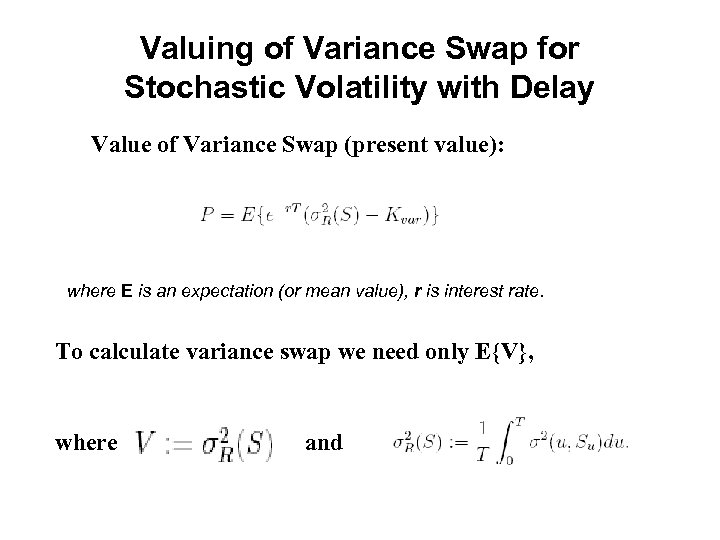Valuing of Variance Swap for Stochastic Volatility with Delay Value of Variance Swap (present value): where E is an expectation (or mean value), r is interest rate. To calculate variance swap we need only E{V}, where and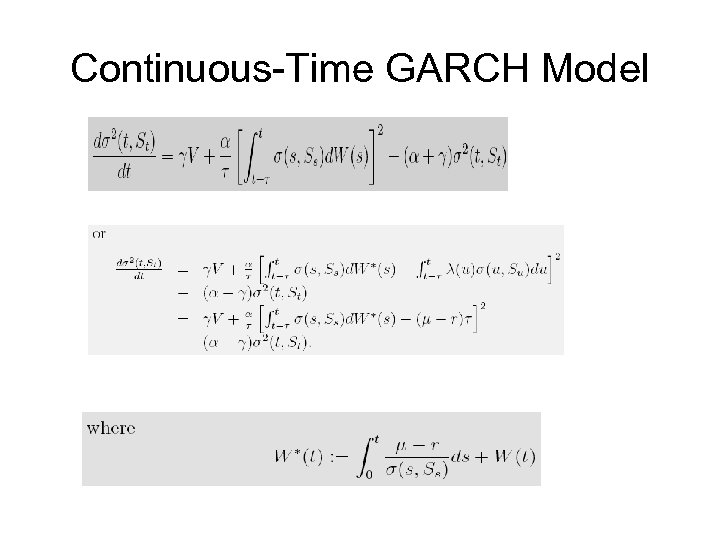Continuous-Time GARCH Model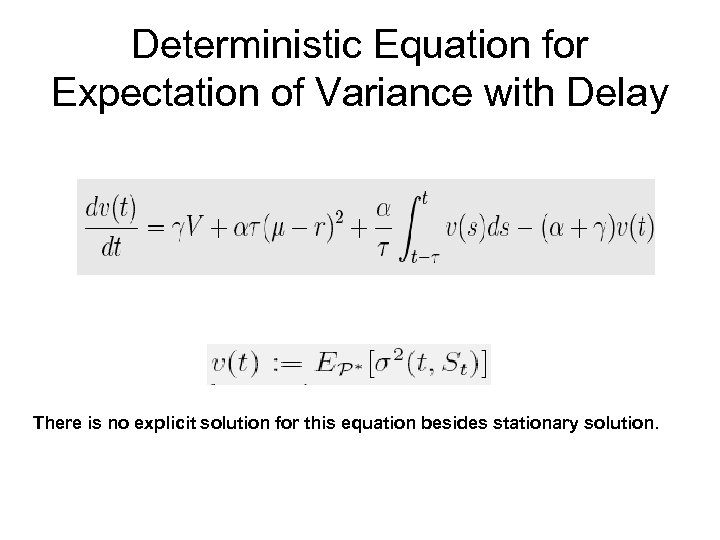Deterministic Equation for Expectation of Variance with Delay There is no explicit solution for this equation besides stationary solution.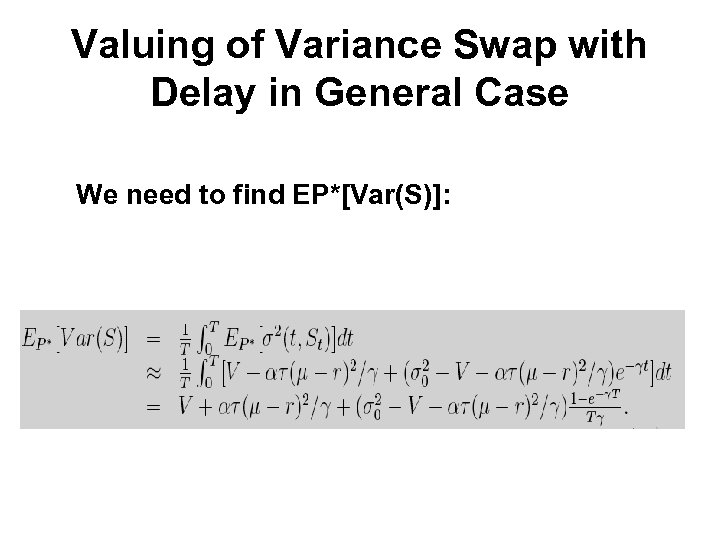Valuing of Variance Swap with Delay in General Case We need to find EP*[Var(S)]: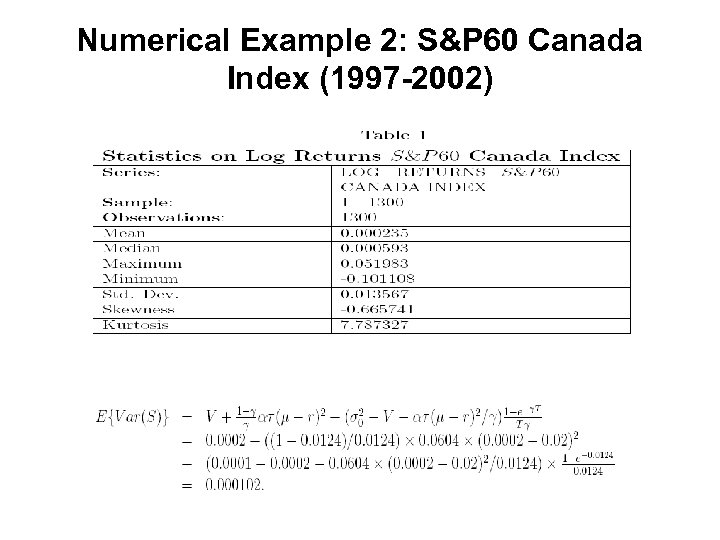Numerical Example 2: S&P 60 Canada Index (1997 -2002)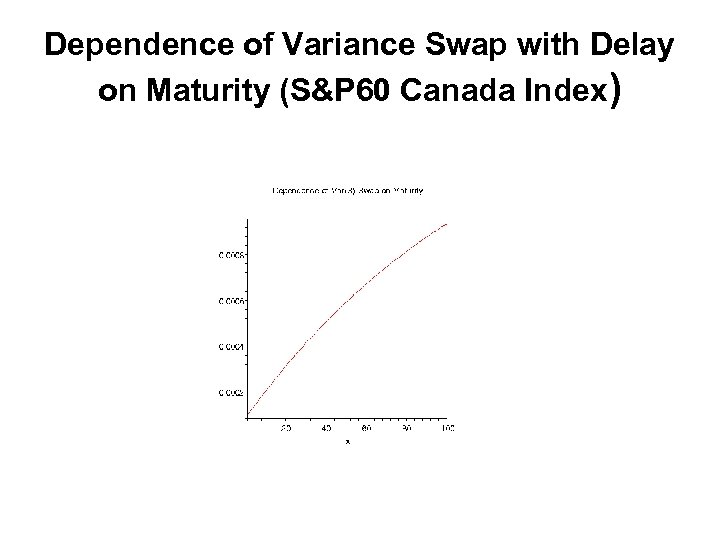Dependence of Variance Swap with Delay on Maturity (S&P 60 Canada Index)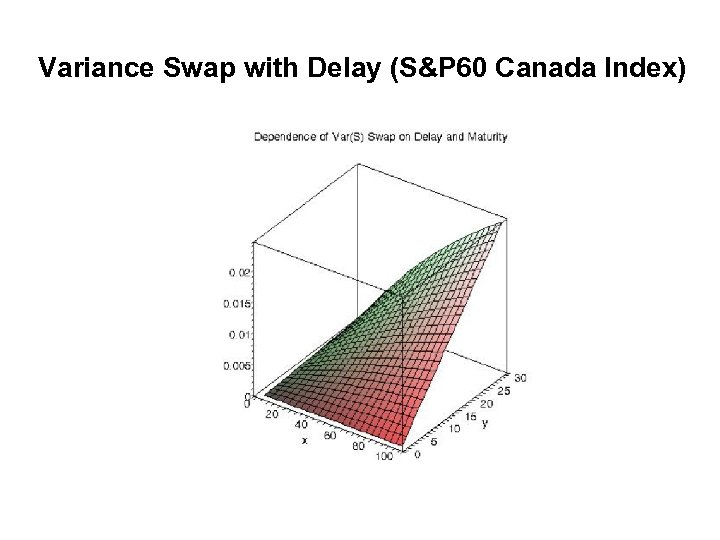Variance Swap with Delay (S&P 60 Canada Index)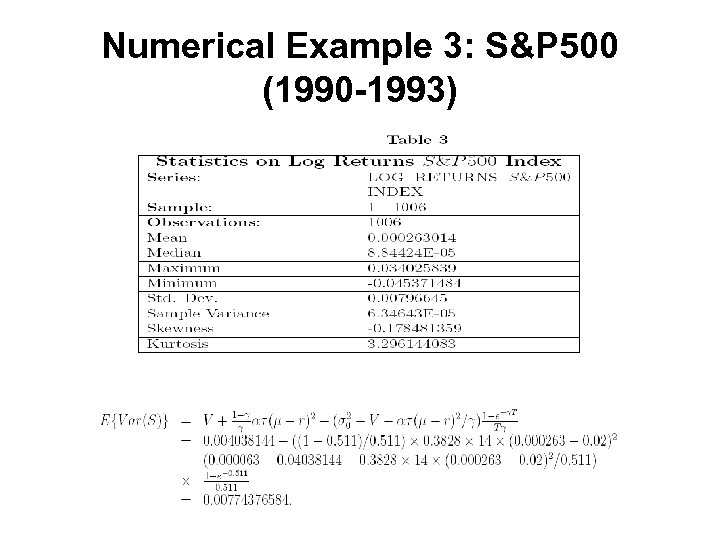Numerical Example 3: S&P 500 (1990 -1993)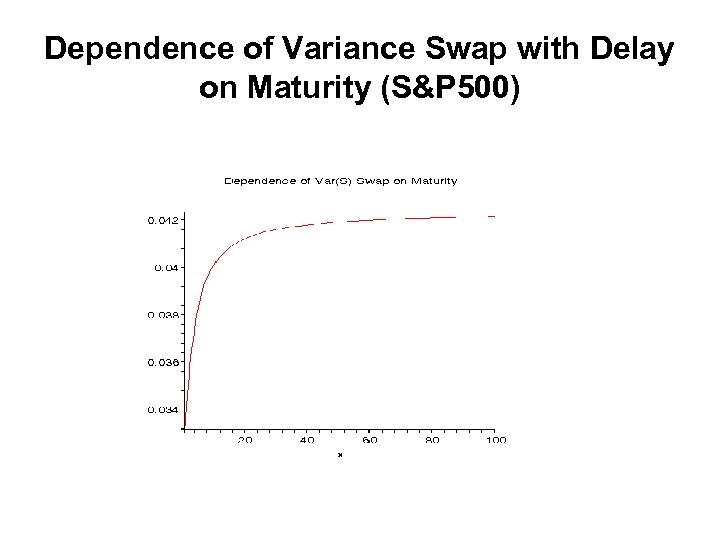Dependence of Variance Swap with Delay on Maturity (S&P 500)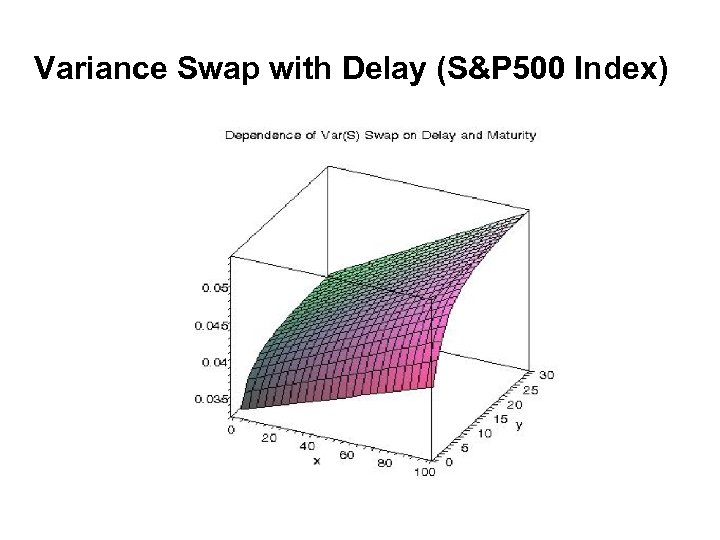Variance Swap with Delay (S&P 500 Index)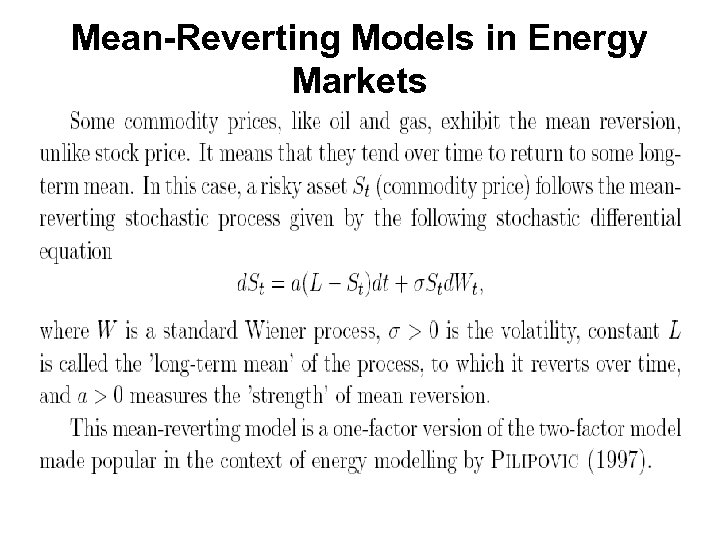Mean-Reverting Models in Energy Markets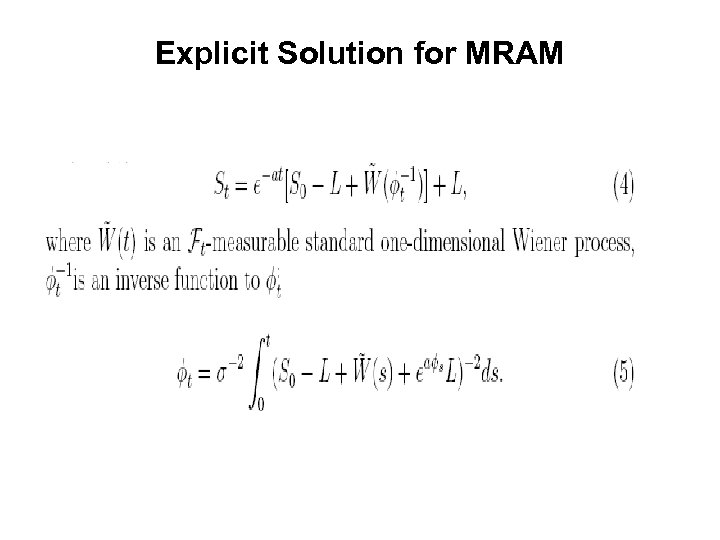Explicit Solution for MRAM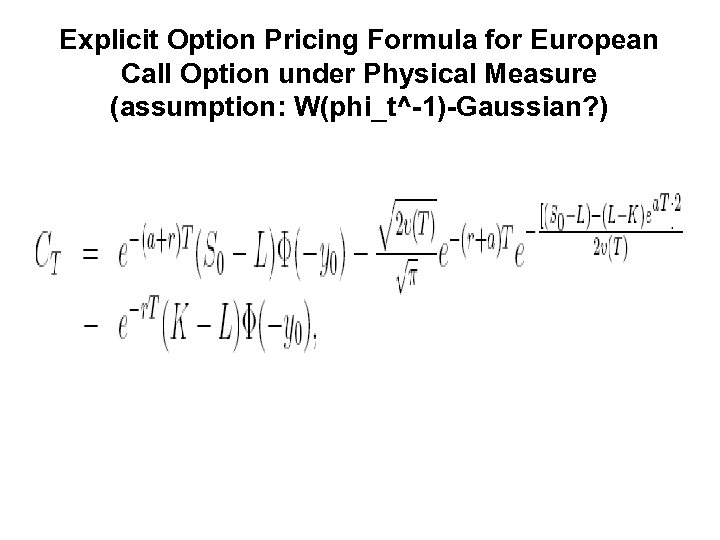Explicit Option Pricing Formula for European Call Option under Physical Measure (assumption: W(phi_t^-1)-Gaussian? )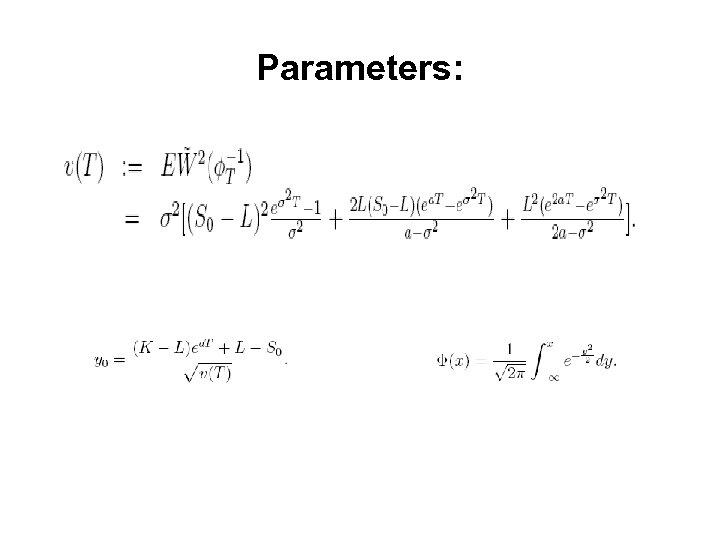Parameters: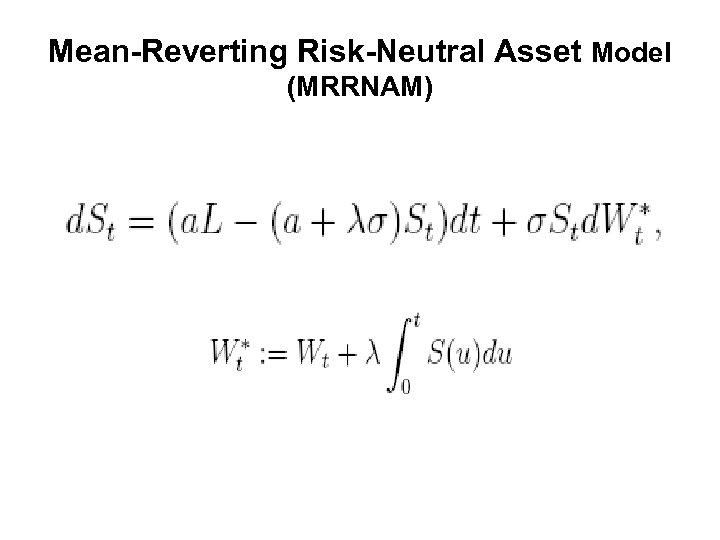Mean-Reverting Risk-Neutral Asset Model (MRRNAM)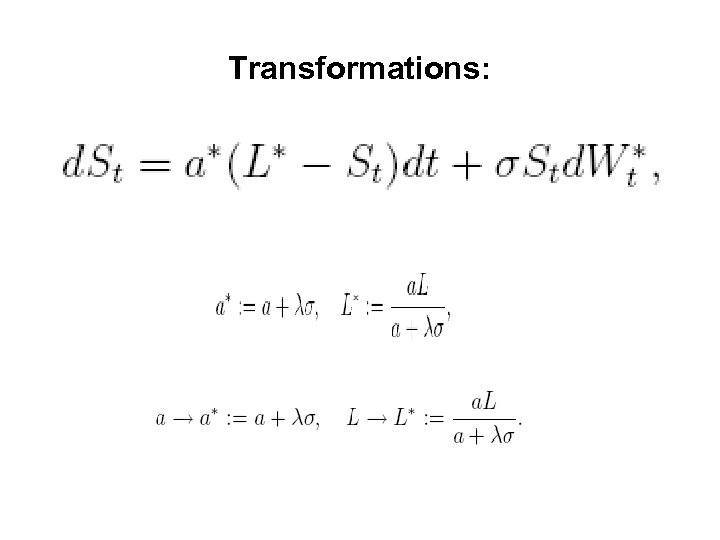Transformations: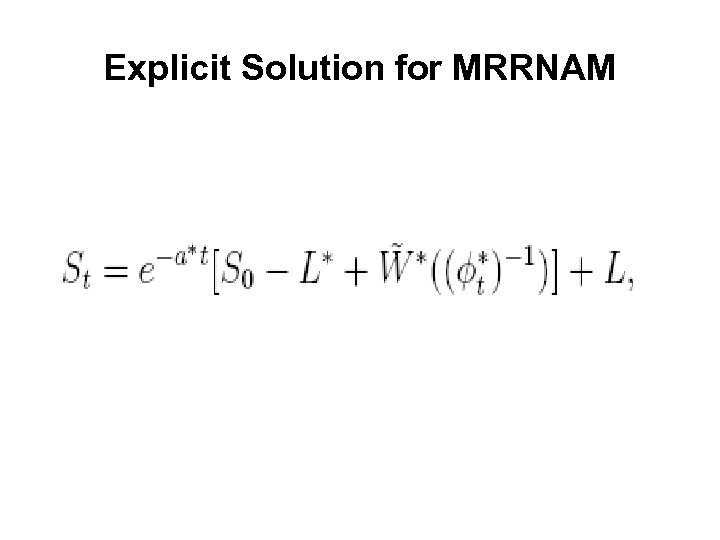Explicit Solution for MRRNAM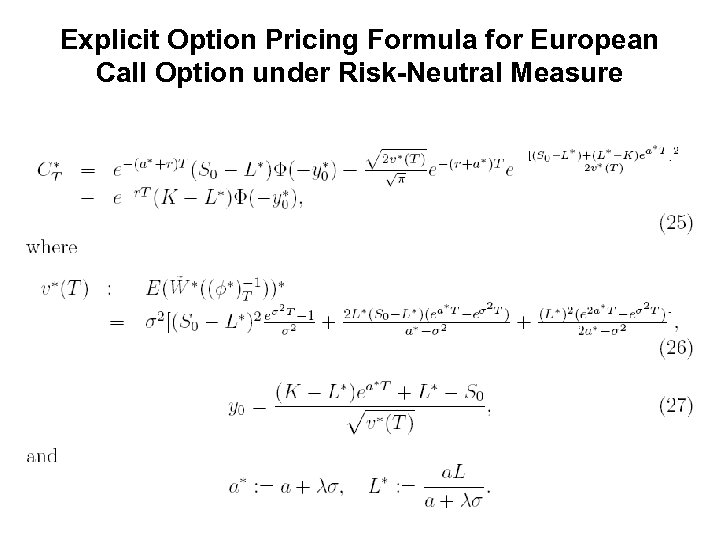Explicit Option Pricing Formula for European Call Option under Risk-Neutral Measure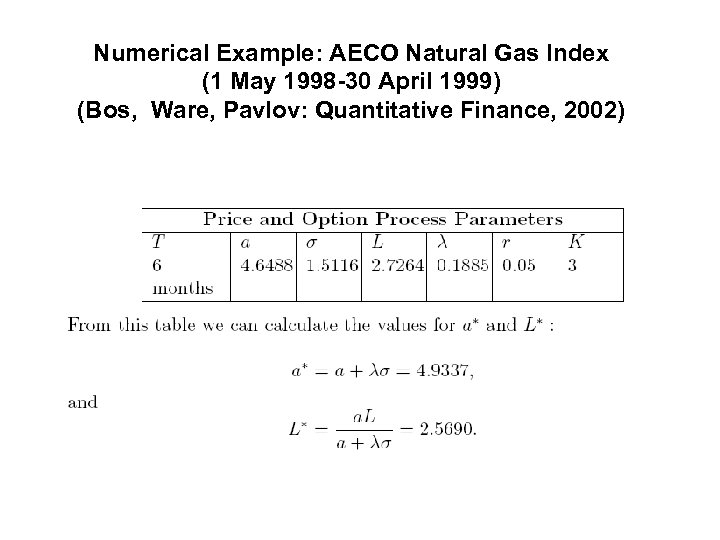Numerical Example: AECO Natural Gas Index (1 May 1998 -30 April 1999) (Bos, Ware, Pavlov: Quantitative Finance, 2002)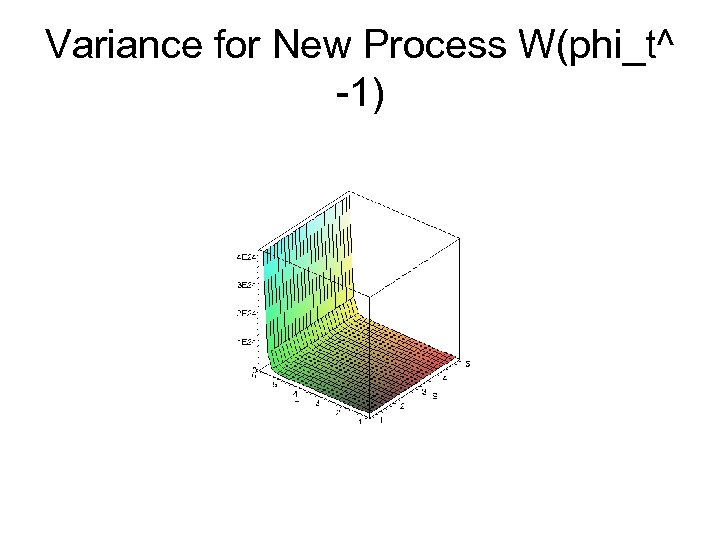Variance for New Process W(phi_t^ -1)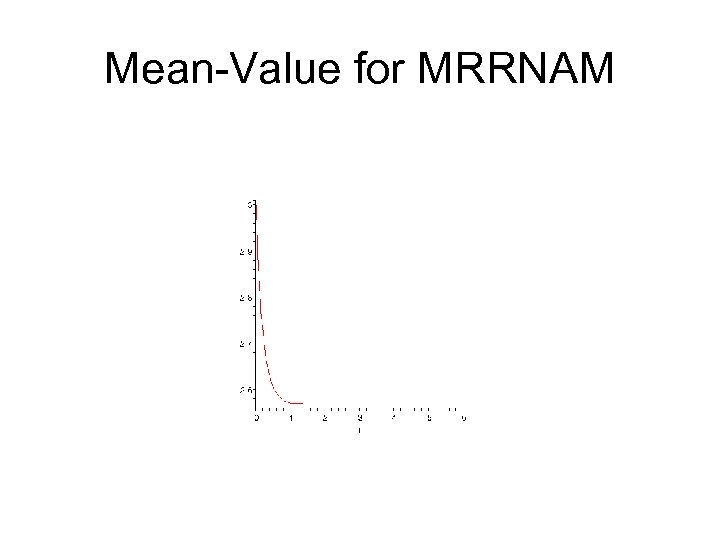Mean-Value for MRRNAM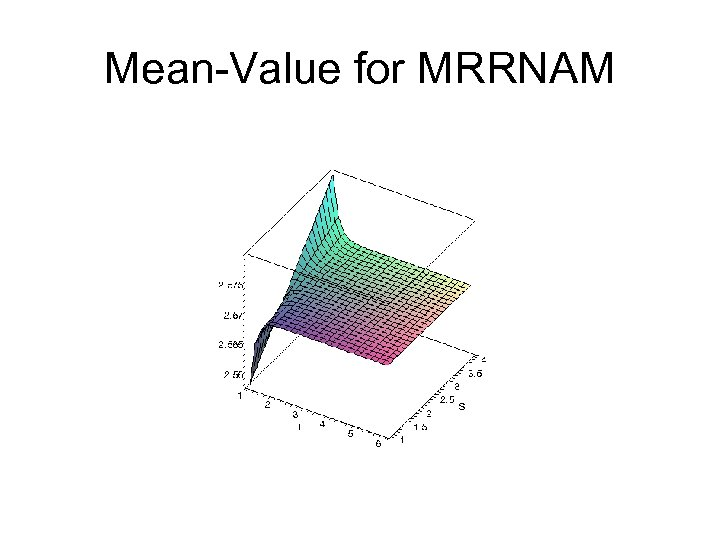Mean-Value for MRRNAM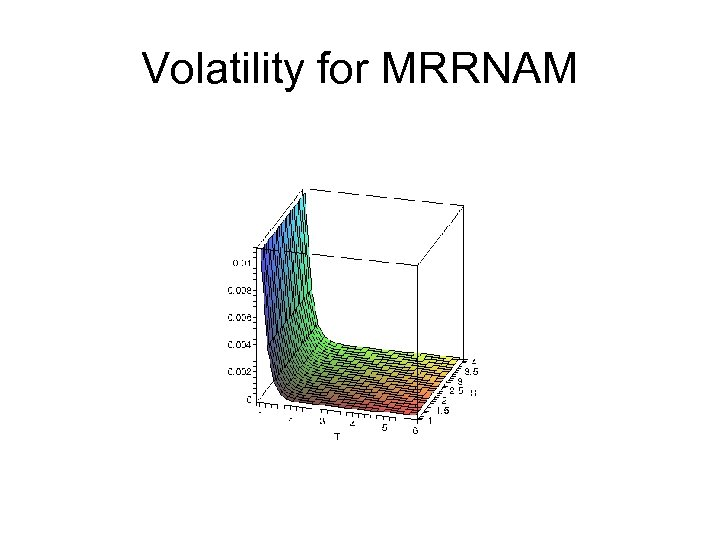Volatility for MRRNAM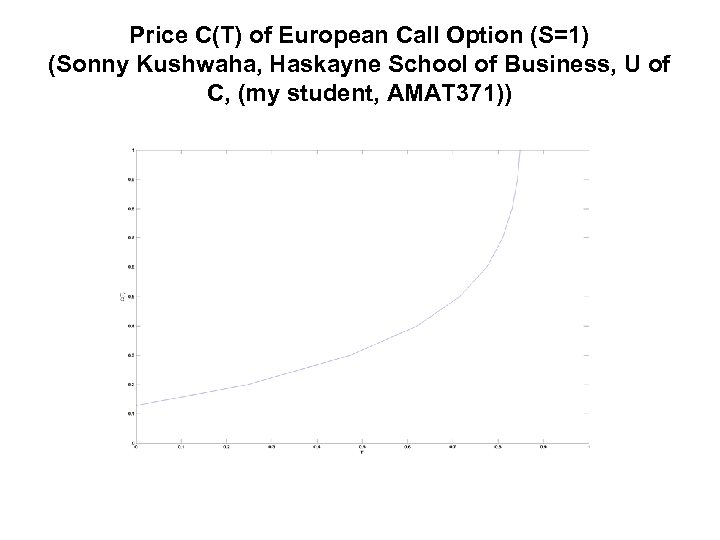Price C(T) of European Call Option (S=1) (Sonny Kushwaha, Haskayne School of Business, U of C, (my student, AMAT 371))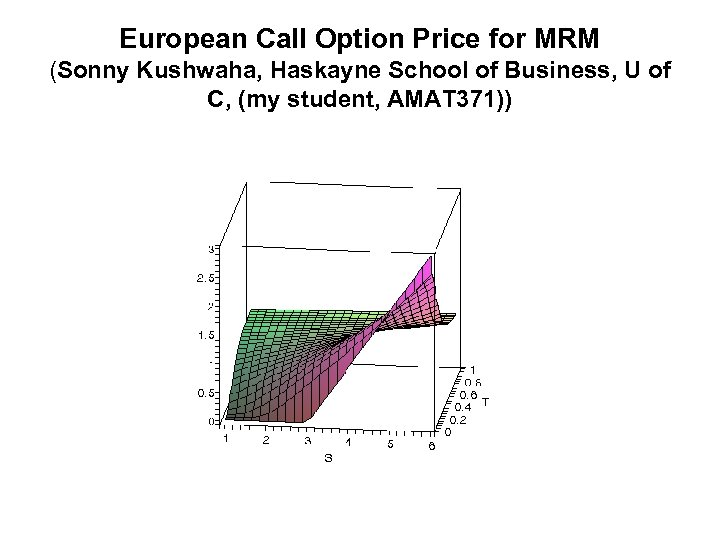European Call Option Price for MRM (Sonny Kushwaha, Haskayne School of Business, U of C, (my student, AMAT 371))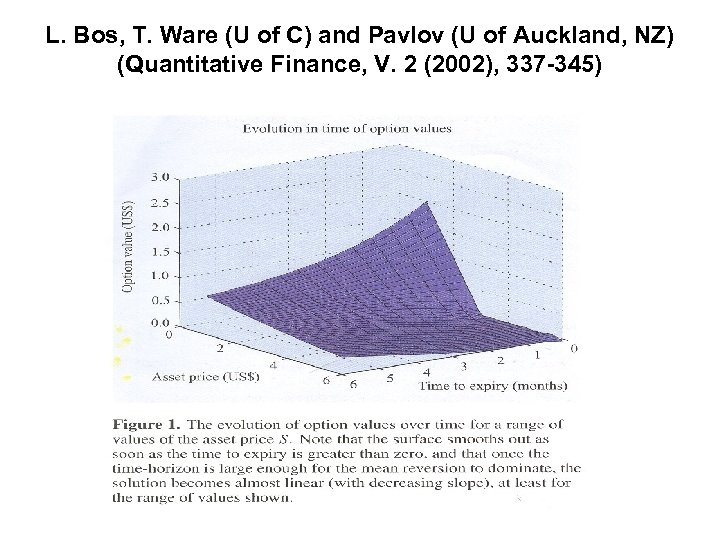L. Bos, T. Ware (U of C) and Pavlov (U of Auckland, NZ) (Quantitative Finance, V. 2 (2002), 337 -345)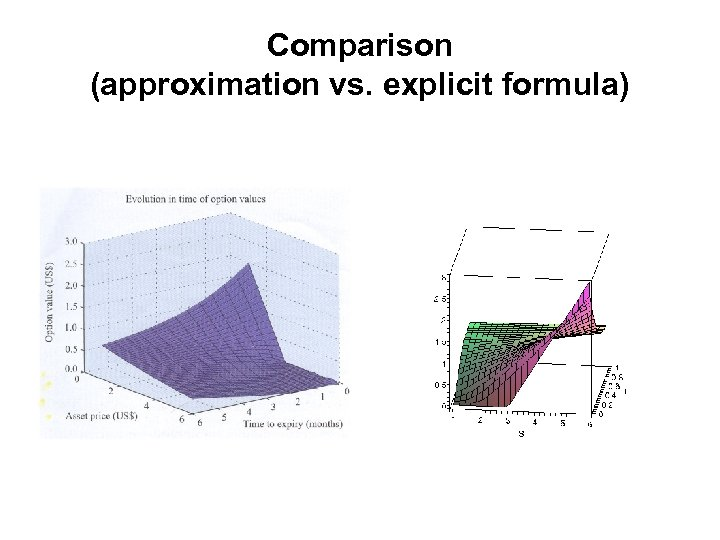Comparison (approximation vs. explicit formula)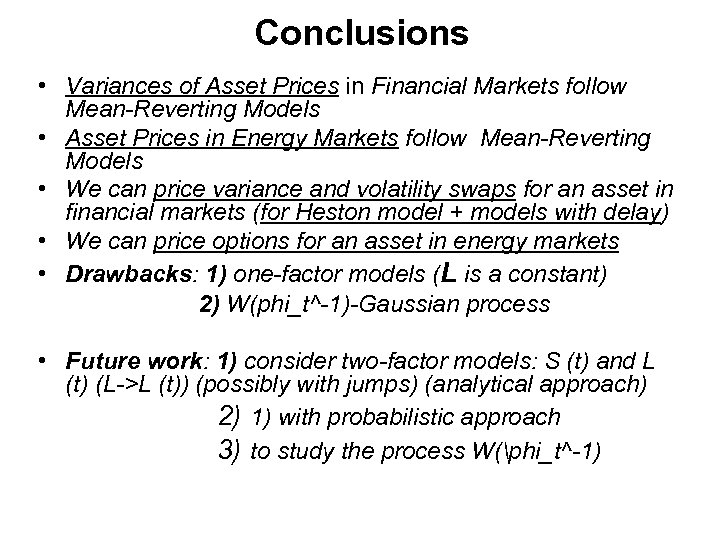Conclusions • Variances of Asset Prices in Financial Markets follow Mean-Reverting Models • Asset Prices in Energy Markets follow Mean-Reverting Models • We can price variance and volatility swaps for an asset in financial markets (for Heston model + models with delay) • We can price options for an asset in energy markets • Drawbacks: 1) one-factor models (L is a constant) 2) W(phi_t^-1)-Gaussian process • Future work: 1) consider two-factor models: S (t) and L (t) (L->L (t)) (possibly with jumps) (analytical approach) 2) 1) with probabilistic approach 3) to study the process W(phi_t^-1)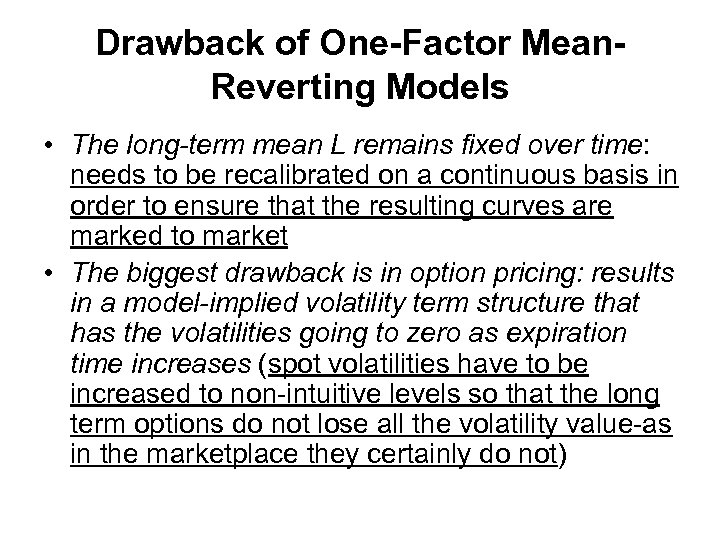Drawback of One-Factor Mean. Reverting Models • The long-term mean L remains fixed over time: needs to be recalibrated on a continuous basis in order to ensure that the resulting curves are marked to market • The biggest drawback is in option pricing: results in a model-implied volatility term structure that has the volatilities going to zero as expiration time increases (spot volatilities have to be increased to non-intuitive levels so that the long term options do not lose all the volatility value-as in the marketplace they certainly do not)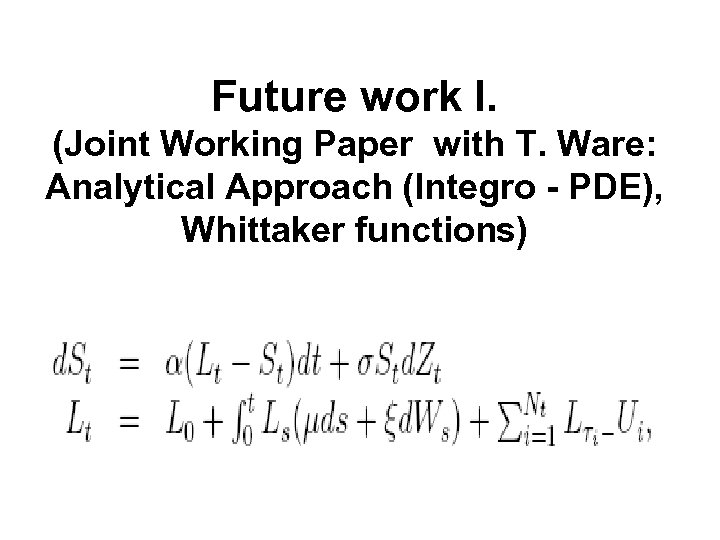Future work I. (Joint Working Paper with T. Ware: Analytical Approach (Integro - PDE), Whittaker functions)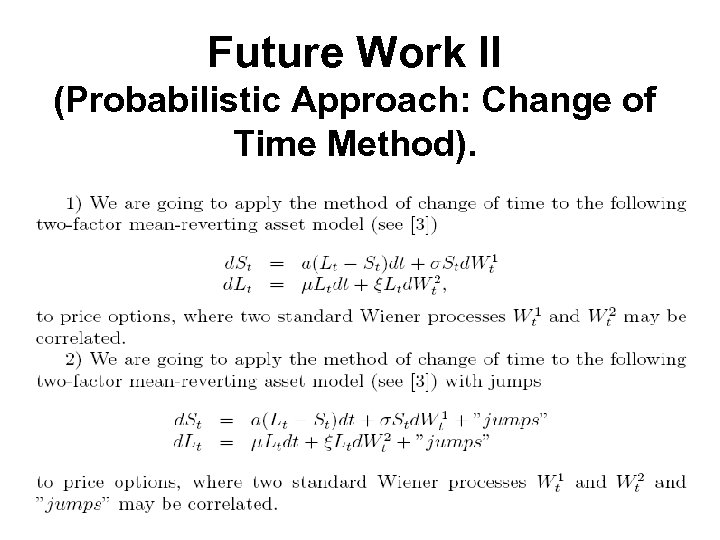Future Work II (Probabilistic Approach: Change of Time Method).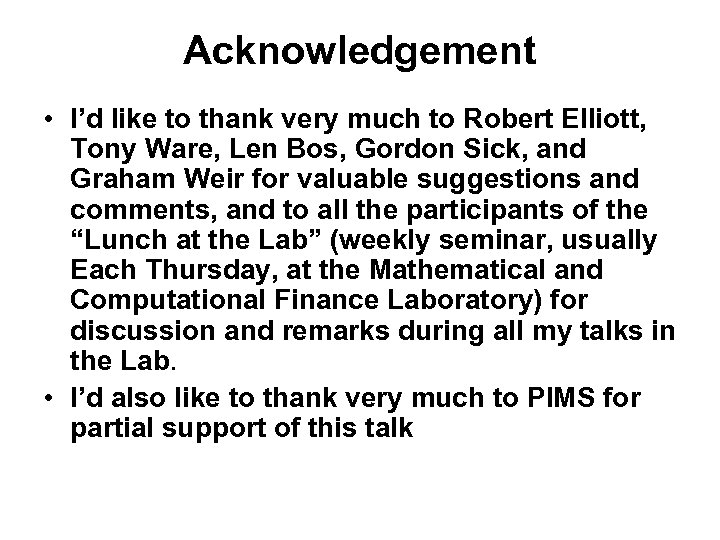Acknowledgement • I’d like to thank very much to Robert Elliott, Tony Ware, Len Bos, Gordon Sick, and Graham Weir for valuable suggestions and comments, and to all the participants of the “Lunch at the Lab” (weekly seminar, usually Each Thursday, at the Mathematical and Computational Finance Laboratory) for discussion and remarks during all my talks in the Lab. • I’d also like to thank very much to PIMS for partial support of this talkThank you for your attention!